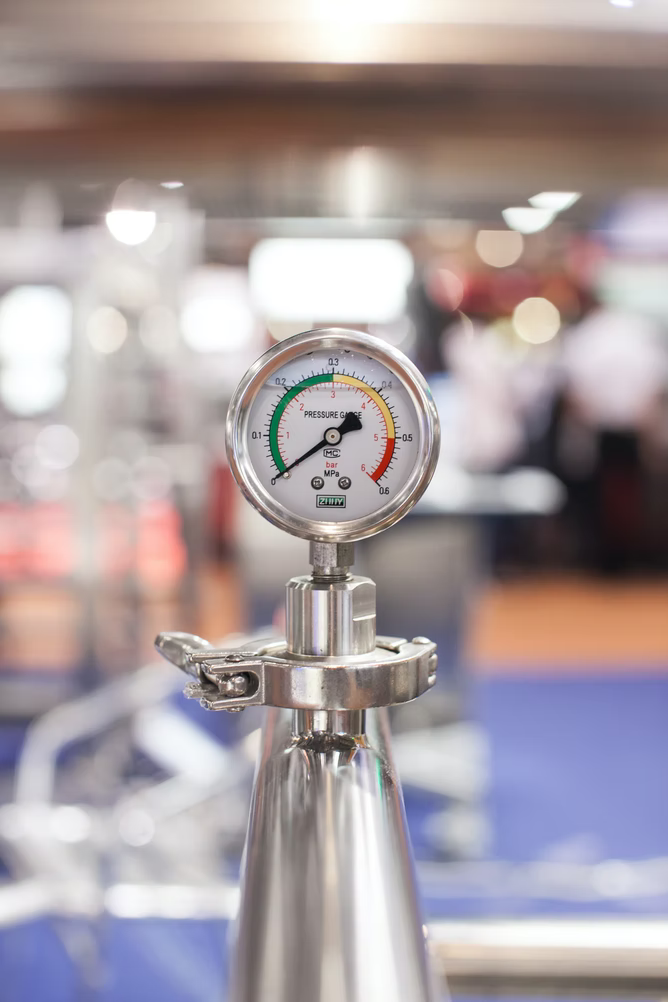## Pressure Units

What are the base SI units for pressure?Hint
Pressure is a force applied perpendicularly over an area. The unit for pressure is a pascal (Pa).
$$Pressure=\frac{Force}{Area}$$$Hint 2 A pascal is: $$1\:Pa=1\:\frac{N}{m^2}$$$
where $$N$$ is newton, and $$m$$ is meter.
Pressure is a force applied perpendicularly over an area. The unit for pressure is a pascal (Pa), which is one newton (N) per square meter.
$$1\:Pa=1\:\frac{N}{m^2}$$$A newton is a unit of force, which is described in the following SI base units: $$\frac{m\cdot kg}{s^{2}}$$$
Combining all units together:
$$Pa=\frac{N}{m^2}=\frac{kg}{m\cdot s^2}$$$$$\frac{kg}{m\cdot s^2}$$$